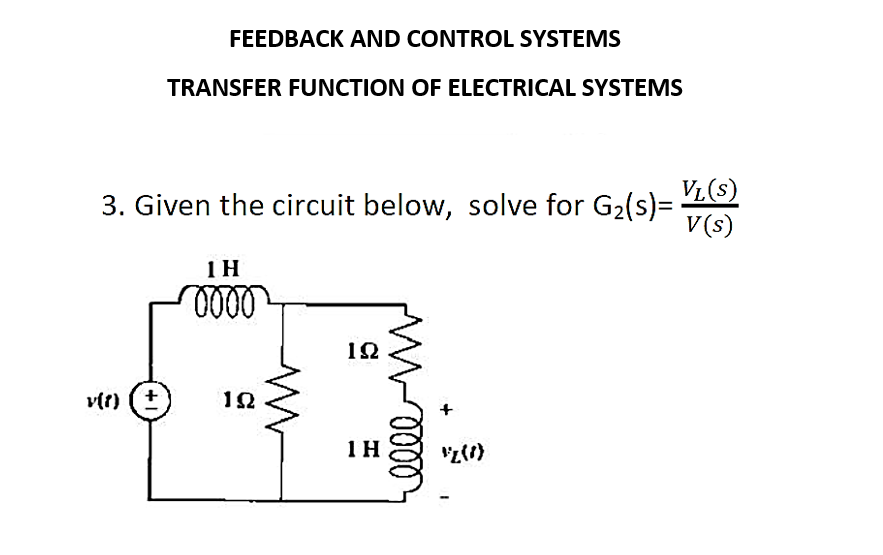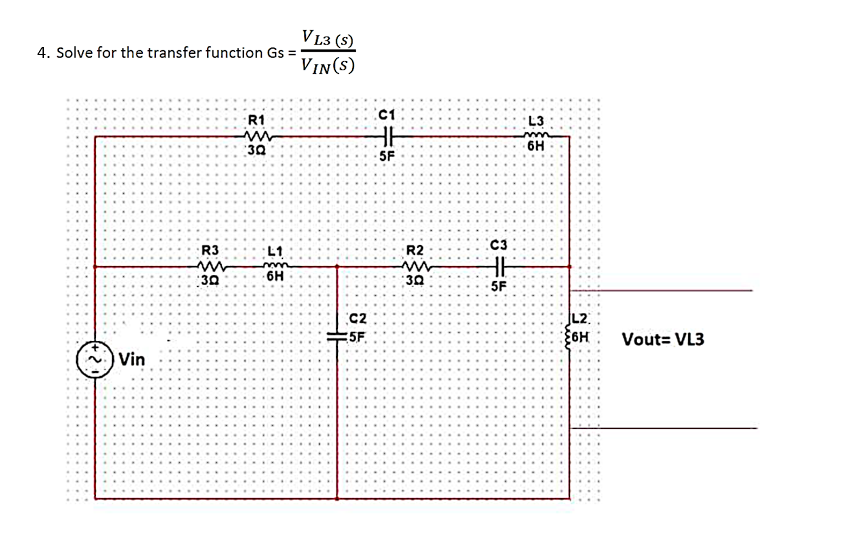# Question please help me to solve this. please show complete solutions FEEDBACK AND CONTROL SYSTEMS TRANSFER FUNCTION OF ELECTRICAL SYSTEMS 3. Given the circuit below, solve for $$\mathrm{G}_{2}(\mathrm{~s})=\frac{V_{L}(s)}{V(s)}$$ $$\mathrm{Gs}=\frac{V_{L 3}(s)}{V_{I N}(s)}$$D5TVJ3 The Asker · Electrical EngineeringTranscribed Image Text: FEEDBACK AND CONTROL SYSTEMS TRANSFER FUNCTION OF ELECTRICAL SYSTEMS 3. Given the circuit below, solve for $$\mathrm{G}_{2}(\mathrm{~s})=\frac{V_{L}(s)}{V(s)}$$ $$\mathrm{Gs}=\frac{V_{L 3}(s)}{V_{I N}(s)}$$
Transcribed Image Text: FEEDBACK AND CONTROL SYSTEMS TRANSFER FUNCTION OF ELECTRICAL SYSTEMS 3. Given the circuit below, solve for $$\mathrm{G}_{2}(\mathrm{~s})=\frac{V_{L}(s)}{V(s)}$$ $$\mathrm{Gs}=\frac{V_{L 3}(s)}{V_{I N}(s)}$$&#12304;General guidance&#12305;The answer provided below has been developed in a clear step by step manner.Step1/2Given circuit, <path transform="" transform-origin="135 225" d=" M 140 225 A 5 5 0 1,0 145 230 A 5 5 0 0,0 140 225 " fill="transparent" stroke="transparent" stroke-width="2" stroke-dasharray="0" stroke-linecap="round" stroke-linejoin="round" stroke-miterlimit="10" dominant-baseline="text-before-edge" vector-effect="non-scaling-stroke" data-id="connection-anchor-15QArW">v(t)1 H1 ohm1 ohm1 H+-vL(t)To find the transfer function $$\mathrm{\frac{{{V}_{{L}}{\left({s}\right)}}}{{{V}{\left({s}\right)}}}}$$Explanation:Transfer function is the ratio of the output voltage to the input voltage of the system.Explanation:Please refer to solution in this step.Step2/2First, convert the circuit from the time domain to s-domain,<path transform="" transform-origin="155 245" d=" M 160 245 A 5 5 0 1,0 165 250 A 5 5 0 0,0 160 245 " fill="transparent" stroke="transparent" stroke-width="2" stroke-dasharray="0" stroke-linecap="round" stroke-linejoin="round" stroke-miterlimit="10" dominant-baseline="text-before-edge" vector-effect="non-scaling-stroke" data-id="connection-anchor-klQLCc">V(s)1s1 ohm1 ohm1s+-V_L(s)I1(s)I2(s)Explanation:Inductor in s-domain is the product of the inductor with s.Applying mesh analysis at the loop 1,\( \begin{align*} \mathrm{{V}{\left({s}\right)}-{1}{s}{I}_{{1}}{\left({s}\right)}-{1}{\left({I}_{{1}}{\left({s}\right)}-{ ... See the full answer